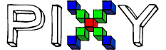# X,y coordinates

hello,
I am fairly new to pixy cam and Arduino but I wanted to know if my statement is valid or not for a project.
by using these commands I want to get the x,y coordinates:
x = pixy.blocks[i].x;
y = pixy.blocks[i].y;
and then use an if statement like this :
if(0 < x && x < 105 && y < 207 && 138 < y)

is what I’m doing going to work?
(pixy will be fixed and will get data from a moving object then move motors)

Hello,
You are missing some things like pixy.ccc.blocks and the for loop, but the general structure looks goodYou can also refer to the ccc_hello_world code and the API for some general guidelines:

https://docs.pixycam.com/wiki/doku.php?id=wiki:v2:ccc_api

Edward

I just wanted to make sure of what you mean so I adjusted the code and here is a sample of it (for pixy), I would appreciate if you would take a look it.

#include <AFMotor.h>
#include <SPI.h>
#include <Pixy2.h>

Pixy2 pixy;

int signature = 0;
int x = 0; //positon x axis
int y = 0; //position y axis
int Xmin = 0; //min x position
int Xmax = 315; //max x position
int Ymin = 0; //min y position
int Ymax = 207; //max y position
static int i = 0;

void setup() {
Serial.begin(115200);
pixy.init();
}

void loop() {
while(millis()<5000)
{
scan();
}

``````scan();
``````

if(signature ==1 )//looking for signature 1
{
if (Xmin < x && x < 105 && y < Ymax && 138 < y) //go downleft if (0 < x < 105 , 138 < y < 207)
{
downleft();
}
}
void scan()
{
uint16_t blocks;
blocks = pixy.ccc.getBlocks(); //receive data from pixy
signature = pixy.ccc.blocks[i].m_signature; //get object’s signature
x = pixy.ccc.blocks[i].m_x; //get x position
y = pixy.ccc.blocks[i].m_y; //get y position
}

Hello,
I’m hesitant to turn this forum into a C coding workshop. Your interaction with the Pixy API looks sound, but you have issues with looping (for example, check your i variable, how it’s incremented, it’s limits, etc.) Have someone who is more familiar with C help, and leverage the example code (ccc_hello_world).

Hope this helps.

Edward

2 Likes